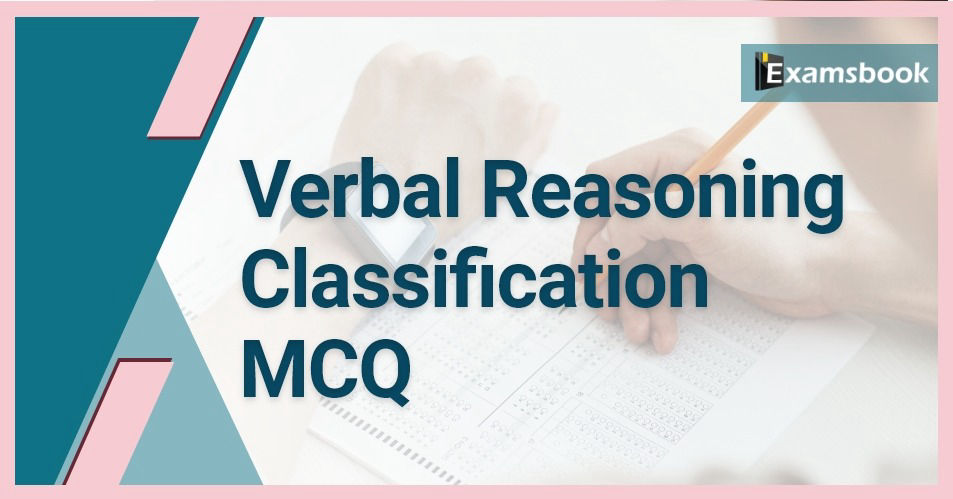• Save

# Verbal Reasoning Classification MCQ QuestionsHere we are providing Classification important questions and answers based on the above formulas for your convenience and good practice will help you to improve your score by covering the maths topic. Students who understand mathematics as a very difficult subject, start their practice -

## Verbal Reasoning Classification MCQ Questions

Q :

Four numbers have been given out of which three are alike in some manner, while one is different. Choose the odd one.

(A) 91

(B) 73

(C) 109

(D) 61

Q :

Select the option that belongs to the category of words given below.
Cinema, Actor, Director

(A) Book

(B) Disease

(C) Principal

(D) Theater

Q :

Four words have been given out of which three are alike in some manner, while one is different .Choose the odd one.

(A) Cut

(B) knife

(C) Scalpel

(D) Chisel

Q :

Find the odd one.

(A) Ginger

(B) Garlic

(C) Chili

(D) Potato

Q :

Four number have been given out of which three are alike in some manner, while is different. Choose the odd one.

(A) 91

(B) 196

(C) 169

(D) 126

Q :

Find the odd word in the following options.

(A) 4913

(B) 2910

(C) 1331

(D) 6859

Q :

Four words have been given out of which three are alike in some manner, while one is different. Choose the odd one.

(A) Money

(B) Lira

(C) Rial

(D) Dollar

Q :

Find the odd word in the following options.

(A) DHJ

(B) MQS

(C) JHN

(D) QUW

Q :

Four pairs of letter-cluster have been given out of which three are alike in some manner, while one is different. Choose the odd one.

(A) NVH-KUK

(B) REA-ODD

(C) CWX-AVZ

(D) TXB-QWE

Q :

Find the odd word in the following options.

(A) Cow

(B) Goat

(C) Dog

(D) Ox